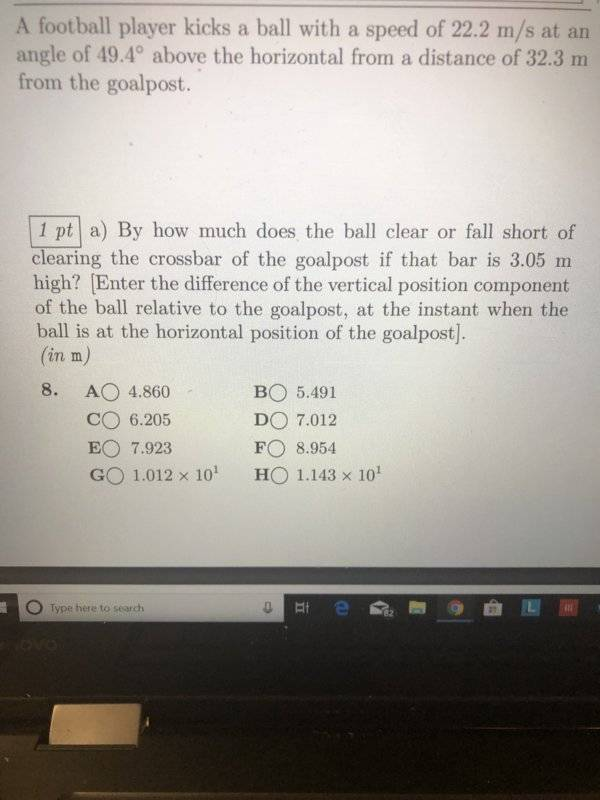# Kinematics of a kicked football

Josh0768
Homework Statement:
Please help, I am thoroughly stumped trying to solve this kinematics question on my study guide. I’ve tried everything I know of but cannot get an answer that’s even an option.
Relevant Equations:
R = v^2sin(2theta)/g
v^2 = vi^2 -2gd
x = vcos(theta)t•Delta2

Homework Helper
x = vcos(theta)t
You could solve that equation for the time it takes to reach the horizontal position of the goal post.
It seems to me that you have forgotten to write down the equation for the vertical position of a ballistic object as a function of time.

•Delta2
Homework Helper
Gold Member
2022 Award
I’ve tried everything I know
I assume you would like us to tell you where you are going wrong. Just a moment while I get out my crystal ball...

•berkeman and Delta2
Homework Helper
Hello Josh.

How are you going to solve this problem without the equation for vertical position as a function of time? If you can figure out the elapsed time when the football is at the required height (3.05 m.) I think you can you then solve the rest of the problem.

AM

Nimarjeet Bajwa
Homework Statement: Please help, I am thoroughly stumped trying to solve this kinematics question on my study guide. I’ve tried everything I know of but cannot get an answer that’s even an option.
Homework Equations: R = v^2sin(2theta)/g
v^2 = vi^2 -2gd
x = vcos(theta)t

View attachment 249715
according to me the answer should be "G". Use equation of trajectory and put x=32.3 v=22.2 and theta=49.4 after plugging in the values you should get some "y" after that just subtract the height of the goal post.